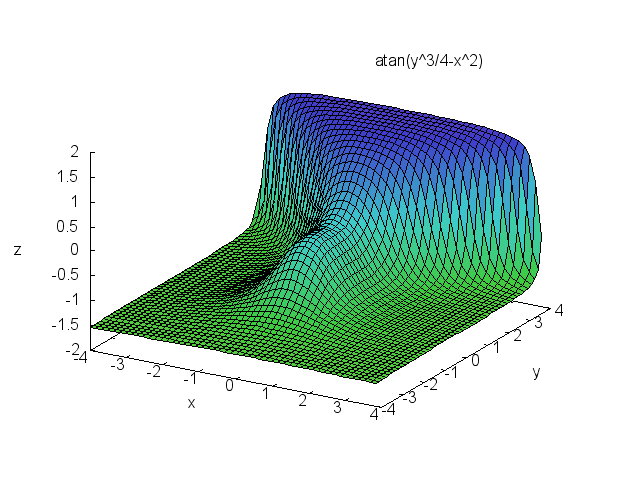Support via Liberapay, GitHub or PayPal

# Maxima Source Code Blocks in Org Mode

Org Mode support for Maxima

## Introduction

Maxima is a computer algebra system descended from Macsyma, which was originally released in 1982. It is released under the terms of the GNU General Public License.

Maxima is written in Common Lisp. It can be accessed and extended in Lisp.

## Requirements and Setup

Maxima binaries are available for Windows and Linux. These either require a compatible Common Lisp or an executable Lisp image. Mac OS X users can install via brew or MacPorts. The combinations of operating system and Lisp implementation known to run Maxima can be found on the Maxima ports page.

There are several versions of Maxima, some with different names. The variable `org-babel-maxima-command` can be set to the name of your Maxima executable. The default value is "maxima".

Maxima ships with `Emacs` modes. Instructions for configuring them can be found on emacswiki. `imaxima` is an `Emacs` front end with image that displays fully typeset math in your `Emacs` window.

You must activate Maxima by adding a line to `org-babel-load-languages`:

```(org-babel-do-load-languages
'((maxima . t))) ; this line activates maxima
```

## Org Mode Features for Maxima Source Code Blocks

There are no Maxima-specific default header argument values.

The header argument, `:cmdline`, can be used to pass command line arguments to Maxima.

### Sessions

Org-mode support for Maxima does not include sessions.

### Result Types

Maxima produces the full range of result types.

## Examples of Use

### Calculator

The following source code block uses `maxima` as a calculator for powers of 12, where the powers are passed with a variable.

```#+name: test-maxima
#+begin_src maxima
programmode: false;
print(12^x);
#+end_src
```
```programmode: false;
print(12^x);
```
```26.06280316745402
```

### Solver

Of course, `maxima` is more than a calculator.

```#+name: solve-maxima
#+begin_src maxima :results output
programmode: false;
eq: x**2-16 = 0;
solution: solve(eq, x);
print(solution);
#+end_src
```
```programmode: false;
eq: x**2-16 = 0;
solution: solve(eq, x);
print(solution);
```

### 3D plots

With `gnuplot` installed (4.0 or higher), 3D graphics are possible. This example is from a tutorial on the maxima/gnuplot interface.

```#+name: 3d-maxima
#+begin_src maxima
programmode: false;
plot3d(atan(-x^2+y^3/4),[x,-4,4],[y,-4,4],[grid,50,50],[gnuplot_pm3d,true]);
#+end_src
```
```programmode: false;
plot3d(atan(-x^2+y^3/4),[x,-4,4],[y,-4,4],[grid,50,50],[gnuplot_pm3d,true]);
```### Inline Display of Maxima LaTeX Output

Maxima code can be evaluated and displayed inline in Org mode through babel 1 as in the example below, based on RS initial example.

```#+NAME: solve-maxima
#+BEGIN_SRC maxima :results raw
tex(exp(-x)/x);
#+END_SRC
```
```tex(exp(-x)/x);
```

#### Toggle inline display of latex code

Latex code in org mode can be displayed inline by 'C-c C-x C-l'. To remove the inline display 'C-c C-c' is used. This is described further in the manual 2.

#### Set scale of output

If the inline display of the equations are illegible, the scale can be set by customising the variable 'org-format-latex-options', by setting the :scale variable to a value >1.

#### Export

This exports nicely to both html (C-c C-e b) and pdf (C-c C-e d). See 3 and 4 in the manual.

#### Noweb expansion

NOTE: I have not tested this yet, but as Eric Schulte noted on the mailing list: "Alternately, if you really want to get fancy you could use noweb expansion 5 to insert the results of the imaxima code block into a latex code block, and then use the existing latex code block functionality to convert the imaxima output to images of different types depending on the export target."

## Footnotes:

1

(info "(org)Library of Babel")

2

(info "(org)Previewing LaTeX fragments")

3

(info "(org)Exporting code blocks")

4

(info "(org)The export dispatcher")

5

(info "(org)noweb")

Documentation from the orgmode.org/worg/ website (either in its HTML format or in its Org format) is licensed under the GNU Free Documentation License version 1.3 or later. The code examples and css stylesheets are licensed under the GNU General Public License v3 or later.